Derivative of logarithmic functions

Derivative of ln x

So far in Calc, we have learned how to find trig derivatives such as the derivative of $\cos x$, and the derivative of $\tan x$. We have also done harder trig derivatives like the derivative of $\sin^{2} x$. Next, we went one step farther and looked at derivatives of inverse trig functions like $\arctan$ and $\arcsin$. Not only that, but we've also looked at differentiating exponential functions like the derivative of $e^{x}$ and even more difficult ones like the derivative of $e^{2x}$. Give yourself a pat in the back because there is only one last derivative to look at now. This will be the derivative of the logarithmic function $\ln x$.

How to Find Derivative of lnx

To find the derivative of $\ln x$, let us start from the very beginning. Consider the equation

•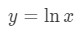Equation 1: Derivative of lnx pt.1
• Note that ln is log with base e, so this can be rewritten as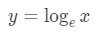Equation 2: Derivative of lnx pt.2
• We need to convert this into exponential form. Doing so will give us the following equation: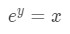Equation 3: Derivative of lnx pt.3
• Let's assume that this is differentiable. Now what we want to do here is something called implicit differentiation. In other words, we want to take the derivative of both sides of the equation. Doing so will give us,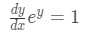Equation 4: Derivative of lnx pt.4
• Notice that dy/dx shows up in the equation because of the chain rule. If you don't understand implicit differentiation or the derivative of exponential functions, we prefer you click those hyperlinks!

• Here is the interesting part. Recall that $y = \ln x$. We can actually substitute y with this in our equation. Doing this will give us: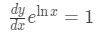Equation 5: Derivative of lnx pt.5
• Now you may think to yourself that we just made the equation even more complicating, but do not worry! The $e^{\ln x}$ in our equation can be simplified to $x$. If you have trouble understanding that, we recommend you to look at the section "Ln as inverse function of exponential function" of this link

http://www.rapidtables.com/math/algebra/Ln.htm
• Since $e^{\ln x} = x$, then we can simply our equation to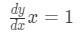Equation 6: Derivative of lnx pt.6
• Lastly we divide both sides of the equation by x, thus giving us the final result: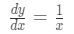Equation 7: Derivative of lnx pt.7
• We're done!

How to Find Second Derivative of lnx

First of all, recall that the first derivative of $\ln x$ is:

•Equation 7: Derivative of lnx pt.7
• Taking the second derivative is not that challenging because we only need to use the quotient rule. Recall that the formula of the quotient rule is:

• The second derivative will be: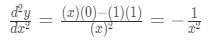Equation 8: Second Derivative of lnx pt.1
• Hence we can conclude that: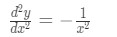Equation 9: Second Derivative of lnx pt.2

Proof of the Derivative of lnx

You may think that finding the derivative of is the same as proving it, but it is actually a little bit different. This is because we cannot assume that $y = \ln x$ is differentiable. Hence we cannot use implicit differentiation like we did earlier. This means that we are going to use a different approach to proving the derivative of $\ln x$.

First of all, consider the following equation:

•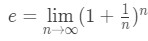Equation 10: Proof of Derivative of lnx pt.1
• We are going to change some variables here and say that $\frac{h}{x} = \frac{1}{n}$ and so $n = \frac{x}{h}$. Notice that for the equation $n = \frac{x}{h}$ as $n$$\infty$, we see that $h$$0$. Hence we can rewrite our formula to be the following: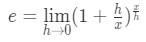Equation 11: Proof of Derivative of lnx pt.2
• Then we can take both sides of the equation to the power of $\frac{1}{x}$, thus giving us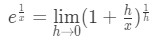Equation 12: Proof of Derivative of lnx pt.3

We will use this formula later in the proof and do a substitution.

• Now to take the derivative of $f(x) = \ln x$, we need to go back to the very beginning and use the definition of derivative. Recall that the definition of derivative is

• If $f(x) = \ln x$, then we will have that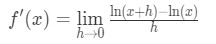Equation 13: Proof of Derivative of lnx pt.4
• Using the logarithm quotient rule, we can change our equation to be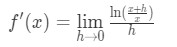Equation 14: Proof of Derivative of lnx pt.5
• Simplifying this equation will give us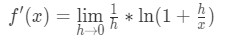Equation 15: Proof of Derivative of lnx pt.6
• We can then use the Logarithm power rule so that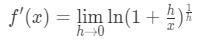Equation 16: Proof of Derivative of lnx pt.7
• Again, if you are not very familiar with logarithm rules, then we suggest you look at the section "Natural logarithm rules and properties" of this link.

http://www.rapidtables.com/math/algebra/Ln.htm

• Now using the property of limits, we can transport the limit so that it is inside the brackets of ln. This will give us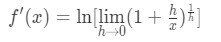Equation 17: Proof of Derivative of lnx pt.8
• Now you might be asking yourself, why does the inside brackets of ln look so familiar? Well that's because earlier we showed that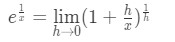Equation 18: Proof of Derivative of lnx pt.9
• So we could actually do a substitution here! Doing so will give us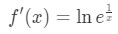Equation 19: Proof of Derivative of lnx pt.10
• Using the Logarithm power rule, we can bring the $\frac{1}{x}$ as a coefficient. This gives usEquation 20: Proof of Derivative of lnx pt.11
• Finally, we know that $\ln e = 1$, so finally we can say that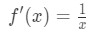Equation 21: Proof of Derivative of lnx pt.12

Derivative of logarithmic functions

A log function is the inverse of an exponential function. In this section, we will learn how to find the derivative of logarithmic functions, including log functions with arbitrary base and natural log functions.

Lessons

$\frac{{d}}{{{d}x}}\;{lo}{{g}_b}\;x = \frac{1}{{x \cdot \;\ln b\;}}$
$\frac{{d}}{{{d}x}}\;{lo}{{g}_b}\left( {\;\;\;\;} \right) = \frac{1}{{\left( {\;\;\;\;} \right)\; \cdot \;\ln b}} \cdot \frac{{d}}{{{d}x}}\left( {\;\;\;\;} \right)$

$\frac{{d}}{{{d}x}}\;{In}x = \frac{1}{x}$
$\frac{{d}}{{{d}x}}\;{In}\left( {\;\;\;\;} \right) = \frac{1}{{\left( {\;\;\;\;} \right)}} \cdot \frac{{d}}{{{d}x}}\left( {\;\;\;\;} \right)$
• 1.
derivative of log with arbitrary base
$\frac{{d}}{{{d}x}}\;{\log _5}x$
$\frac{{d}}{{{d}x}}{\;log}\left( {1000 - \csc x} \right)$

• 2.
derivative of natural log
$\frac{{d}}{{{d}x}}{\;ln}x$
$\frac{{d}}{{{d}x}}{\;ln}\left( {\cos x} \right)$
$\frac{{d}}{{{d}x}}{\;ln}\left( {5{x^3} - 2x + 7} \right)$

• 3.
Differentiate:
$y = {^3}\sqrt{{{\;ln}x\;}}$